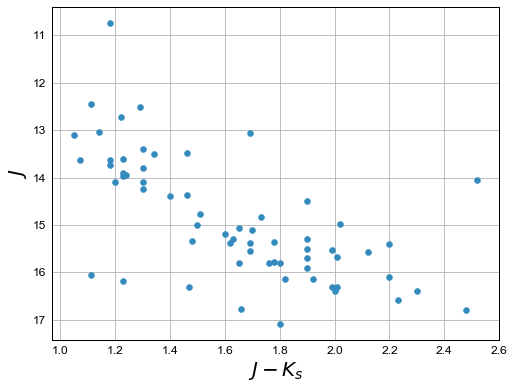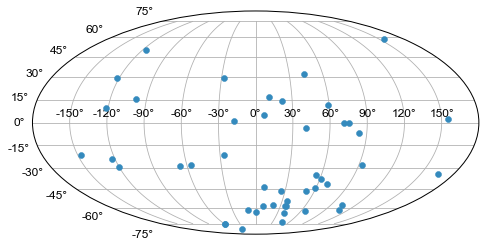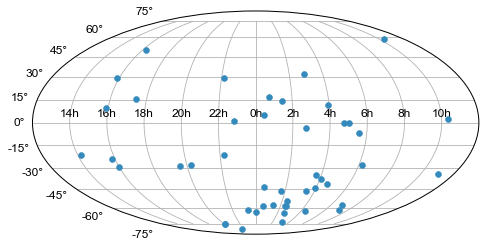In :
import numpy as np

# Set up matplotlib and use a nicer set of plot parameters
%config InlineBackend.rc = {}
import matplotlib
matplotlib.rc_file("../../templates/matplotlibrc")
import matplotlib.pyplot as plt
%matplotlib inline

/Users/erik/miniconda3/envs/astropy-tutorials/lib/python3.5/site-packages/matplotlib/__init__.py:913: UserWarning: axes.color_cycle is deprecated and replaced with axes.prop_cycle; please use the latter.
warnings.warn(self.msg_depr % (key, alt_key))


# Read in catalog information from a text file and plot some parameters¶

Astropy provides functionality for reading in and manipulating tabular data through the astropy.table subpackage. An additional set of tools for reading and writing ASCII data are provided with the astropy.io.ascii subpackage, but fundamentally use the classes and methods implemented in astropy.table.

We'll start by importing the ascii subpackage:

In :
from astropy.io import ascii


For many cases, it is sufficient to use the ascii.read('filename') function as a black box for reading data from table-formatted text files. By default, this function will try to figure out how your data is formatted/delimited (by default, guess=True). For example, if your data are:

# name,ra,dec
BLG100,17:51:00.0,-29:59:48
BLG101,17:53:40.2,-29:49:52
BLG102,17:56:20.2,-29:30:51
BLG103,17:56:20.2,-30:06:22
...



(see _simpletable.csv)

ascii.read() will return a Table object:

In :
tbl = ascii.read("simple_table.csv")
tbl

Out:
<Table length=4>
nameradec
str6str10str9
BLG10017:51:00.0-29:59:48
BLG10117:53:40.2-29:49:52
BLG10217:56:20.2-29:30:51
BLG10317:56:20.2-30:06:22

The header names are automatically parsed from the top of the file, and the delimiter is inferred from the rest of the file -- awesome! We can access the columns directly from their names as 'keys' of the table object:

In :
tbl["ra"]

Out:
<Column name='ra' dtype='str10' length=4>
 17:51:00.0 17:53:40.2 17:56:20.2 17:56:20.2

If we want to then convert the first RA (as a sexagesimal angle) to decimal degrees, for example, we can pluck out the first (0th) item in the column and use the coordinates subpackage to parse the string:

In :
import astropy.coordinates as coord
import astropy.units as u

first_row = tbl # get the first (0th) row
ra = coord.Angle(first_row["ra"], unit=u.hour) # create an Angle object
ra.degree # convert to degrees

Out:
267.75

Now let's look at a case where this breaks, and we have to specify some more options to the read() function. Our data may look a bit messier::

,,,,2MASS Photometry,,,,,,WISE Photometry,,,,,,,,Spectra,,,,Astrometry,,,,,,,,,,,
Name,Designation,RA,Dec,Jmag,J_unc,Hmag,H_unc,Kmag,K_unc,W1,W1_unc,W2,W2_unc,W3,W3_unc,W4,W4_unc,Spectral Type,Spectra (FITS),Opt Spec Refs,NIR Spec Refs,pm_ra (mas),pm_ra_unc,pm_dec (mas),pm_dec_unc,pi (mas),pi_unc,radial velocity (km/s),rv_unc,Astrometry Refs,Discovery Refs,Group/Age,Note
,00 04 02.84 -64 10 35.6,1.01201,-64.18,15.79,0.07,14.83,0.07,14.01,0.05,13.37,0.03,12.94,0.03,12.18,0.24,9.16,null,L1γ,,Kirkpatrick et al. 2010,,,,,,,,,,,Kirkpatrick et al. 2010,,
PC 0025+04,00 27 41.97 +05 03 41.7,6.92489,5.06,16.19,0.09,15.29,0.10,14.96,0.12,14.62,0.04,14.14,0.05,12.24,null,8.89,null,M9.5β,,Mould et al. 1994,,0.0105,0.0004,-0.0008,0.0003,,,,,Faherty et al. 2009,Schneider et al. 1991,,,00 32 55.84 -44 05 05.8,8.23267,-44.08,14.78,0.04,13.86,0.03,13.27,0.04,12.82,0.03,12.49,0.03,11.73,0.19,9.29,null,L0γ,,Cruz et al. 2009,,0.1178,0.0043,-0.0916,0.0043,38.4,4.8,,,Faherty et al. 2012,Reid et al. 2008,,
...



(see Young-Objects-Compilation.csv)

If we try to just use ascii.read() on this data, it fails to parse the names out, and the column names become col followed by the number of the column:

In :
tbl = ascii.read("Young-Objects-Compilation.csv")
tbl.colnames

Out:
['col1',
'col2',
'col3',
'col4',
'col5',
'col6',
'col7',
'col8',
'col9',
'col10',
'col11',
'col12',
'col13',
'col14',
'col15',
'col16',
'col17',
'col18',
'col19',
'col20',
'col21',
'col22',
'col23',
'col24',
'col25',
'col26',
'col27',
'col28',
'col29',
'col30',
'col31',
'col32',
'col33',
'col34']

What happened? The column names are just col1, col2, etc., the default names if ascii.read() is unable to parse out column names. We know it failed to read the column names, but also notice that the first row of data are strings -- something else went wrong!

In :
tbl

Out:
<Row index=0 masked=True>
col1col2col3col4col5col6col7col8col9col10col11col12col13col14col15col16col17col18col19col20col21col22col23col24col25col26col27col28col29col30col31col32col33col34
str24str25str9str6str16str5str5str5str5str5str15str6str5str6str5str6str4str6str13str14str26str23str11str9str12str10str8str6str22str6str19str23str9str38
--------2MASS Photometry----------WISE Photometry--------------Spectra------Astrometry----------------------

A few things are causing problems here. First, there are two header lines in the file and the header lines are not denoted by comment characters. The first line is actually some meta data that we don't care about, so we want to skip it. We can get around this problem by specifying the header_start keyword to the ascii.read() function. This keyword argument specifies the index of the row in the text file to read the column names from:

In :
tbl = ascii.read("Young-Objects-Compilation.csv", header_start=1)
tbl.colnames

Out:
['Name',
'Designation',
'RA',
'Dec',
'Jmag',
'J_unc',
'Hmag',
'H_unc',
'Kmag',
'K_unc',
'W1',
'W1_unc',
'W2',
'W2_unc',
'W3',
'W3_unc',
'W4',
'W4_unc',
'Spectral Type',
'Spectra (FITS)',
'Opt Spec Refs',
'NIR Spec Refs',
'pm_ra (mas)',
'pm_ra_unc',
'pm_dec (mas)',
'pm_dec_unc',
'pi (mas)',
'pi_unc',
'radial velocity (km/s)',
'rv_unc',
'Astrometry Refs',
'Discovery Refs',
'Group/Age',
'Note']

Great -- now the columns have the correct names, but there is still a problem: all of the columns have string data types, and the column names are still included as a row in the table. This is because by default the data are assumed to start on the second row (index=1). We can specify data_start=2 to tell the reader that the data in this file actually start on the 3rd (index=2) row:

In :
tbl = ascii.read("Young-Objects-Compilation.csv", header_start=1, data_start=2)


Some of the columns have missing data, for example, some of the RA values are missing (denoted by -- when printed):

In :
print(tbl['RA'])

    RA
---------
1.01201
6.92489
8.23267
9.42942
11.33929
--
--
--
21.19163
21.5275
...
300.20171
--
303.46467
321.71
--
--
332.05679
333.43715
342.47273
--
350.72079
Length = 64 rows


This is called a Masked column because some missing values are masked out upon display. If we want to use this numeric data, we have to tell astropy what to fill the missing values with. We can do this with the .filled() method. For example, to fill all of the missing values with NaN's:

In :
tbl['RA'].filled(np.nan)

Out:
<Column name='RA' dtype='float64' length=64>
 1.01201 6.92489 8.23267 9.42942 11.33929 nan nan nan 21.19163 21.5275 25.49263 nan ... 299.19583 300.20171 nan 303.46467 321.71 nan nan 332.05679 333.43715 342.47273 nan 350.72079

Let's recap what we've done so far, then make some plots with the data. Our data file has an extra line above the column names, so we use the header_start keyword to tell it to start from line 1 instead of line 0 (remember Python is 0-indexed!). We then used had to specify that the data starts on line 2 using the data_start keyword. Finally, we note some columns have missing values.

In :
data = ascii.read("Young-Objects-Compilation.csv", header_start=1, data_start=2)


Now that we have our data loaded, let's plot a color-magnitude diagram.

Here we simply make a scatter plot of the J-K color on the x-axis against the J magnitude on the y-axis. We use a trick to flip the y-axis plt.ylim(reversed(plt.ylim())). Called with no arguments, plt.ylim() will return a tuple with the axis bounds, e.g. (0,10). Calling the function with arguments will set the limits of the axis, so we simply set the limits to be the reverse of whatever they were before. Using this pylab-style plotting is convenient for making quick plots and interactive use, but is not great if you need more control over your figures.

In :
plt.scatter(data["Jmag"] - data["Kmag"], data["Jmag"]) # plot J-K vs. J
plt.ylim(reversed(plt.ylim())) # flip the y-axis
plt.xlabel("$J-K_s$", fontsize=20)
plt.ylabel("$J$", fontsize=20)

Out:
<matplotlib.text.Text at 0x111d448d0>As a final example, we will plot the angular positions from the catalog on a 2D projection of the sky. Instead of using pylab-style plotting, we'll take a more object-oriented approach. We'll start by creating a Figure object and adding a single subplot to the figure. We can specify a projection with the projection keyword; in this example we will use a Mollweide projection. Unfortunately, it is highly non-trivial to make the matplotlib projection defined this way follow the celestial convention of longitude/RA increasing to the left.

The axis object, ax, knows to expect angular coordinate values. An important fact is that it expects the values to be in radians, and it expects the azimuthal angle values to be between (-180º,180º). This is (currently) not customizable, so we have to coerce our RA data to conform to these rules! astropy provides a coordinate class for handling angular values, astropy.coordinates.Angle. We can convert our column of RA values to radians, and wrap the angle bounds using this class.

In :
import astropy.coordinates as coord
ra = coord.Angle(data['RA'].filled(np.nan)*u.degree)
ra = ra.wrap_at(180*u.degree)
dec = coord.Angle(data['Dec'].filled(np.nan)*u.degree)

In :
fig = plt.figure(figsize=(8,6))
ax = fig.add_subplot(111, projection="mollweide")
ax.scatter(ra.radian, dec.radian)

Out:
<matplotlib.collections.PathCollection at 0x1121deda0>By default, matplotlib will add degree ticklabels, so let's change the horizontal (x) tick labels to be in units of hours, and display a grid

In :
fig = plt.figure(figsize=(8,6))
ax = fig.add_subplot(111, projection="mollweide")
ax.scatter(ra.radian, dec.radian)
ax.set_xticklabels(['14h','16h','18h','20h','22h','0h','2h','4h','6h','8h','10h'])
ax.grid(True)We can save this figure as a PDF using the savefig function:

In :
fig.savefig("map.pdf")


## Exercises¶

Make the map figures as just above, but color the points by the 'Kmag' column of the table.

In :



Try making the maps again, but with each of the following projections: 'aitoff', 'hammer', 'lambert', and None (which is the same as not giving any projection). Do any of them make the data seem easier to understand?

In :